# ML Aggarwal Class 6 Solutions for ICSE Maths Chapter 2 Whole Numbers Ex 2.2

## ML Aggarwal Class 6 Solutions for ICSE Maths Chapter 2 Whole Numbers Ex 2.2

Question 1.
Fill in the blanks to make each of the following a true statement:
(i) 378 + 1024 = 1024 + …….
(ii) 337 + (528 + 1164) = (337 + ……..) + 1164
(iii) (21 + 18) + ……….. = (21 + 13) + 18
(iv) 3056 + 0 = ……….. = 0 + 3056
Solution: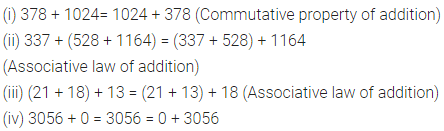Question 2.
Add the following numbers and check by reversing the order of addends :
(i) 3189 + 53885
(ii) 33789 + 50311.
Solution:Question 3.
By suitable arrangements, find the sum of:
(i) 311,528,289
(ii) 723, 834, 66, 277
(iii) 78, 203, 435, 7197, 422.
Solution:Question 4.
Fill in the blanks to make each of the following a true statement:
(i) 375 × 57 = 57 × ……….
(ii) (33 × 16) × 25 = 33 × (…….. × 25)
(iii) 37 × 24 = 37 × 18 + 37 × …………
(iv) 7205 × 1 = …………. = 1 × 7205
(v) 366 × 0 =
(vi) …………… × 579 = 0
(vii) 473 × 108 = 473 × 100 + 473 × ………….
(viii) 684 × 97 = 684 × 100 – …………… × 3
(ix) 0 ÷= 5 =
(x) (14 – 14) ÷ 7 = ………….
Solution: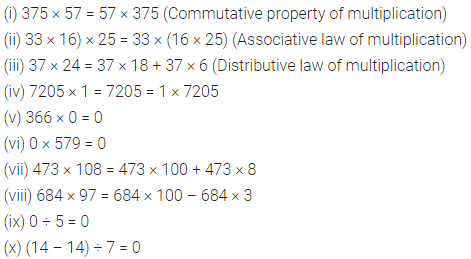Question 5.
Determine the following products by suitable arrangement:
(i) 4 × 528 × 25
(ii) 625 × 239 × 16
(iii) 125 × 40 × 8 × 25
Solution: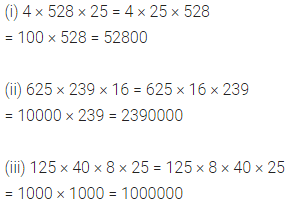Question 6.
Find the value of the following:
(i) 54279 × 92 + 54279 × 8
(ii) 60678 × 262 – 60678 × 162
Solution:Question 7.
Find the following products by using suitable properties:
(i) 739 × 102
(ii) 1938 × 99
(iii) 1005 × 188
Solution: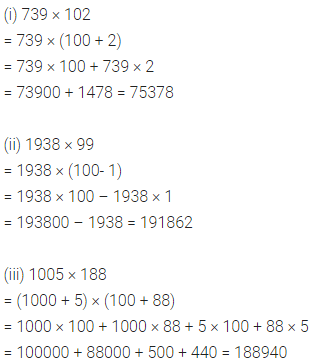Question 8.
Divide 7750 by 17 and check the result by division algorithm.
Solution: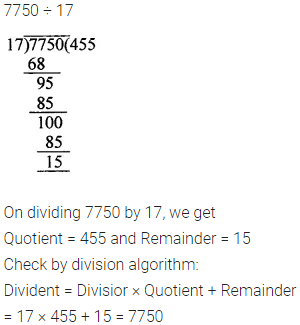Question 9.
Find the number which when divided by 38 gives the quotient 23 and remainder 17.
Solution: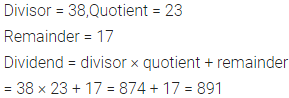Question 10.
Which least number should be subtracted from 1000 so that the difference is exactly divisible by 35.
Solution: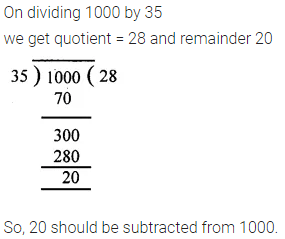Question 11.
Which least number should be added to 1000 so that 53 divides the sum exactly.
Solution: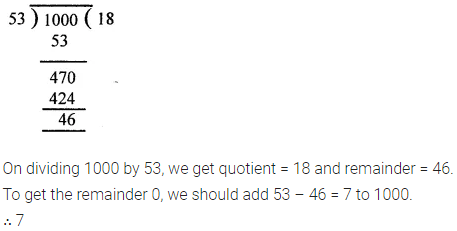Question 12.
Find the largest three-digit number which is exactly divisible by 47.
Solution: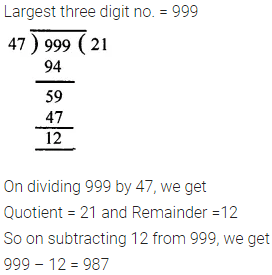Question 13.
Find the smallest five-digit number which is exactly divisible by 254.
Solution: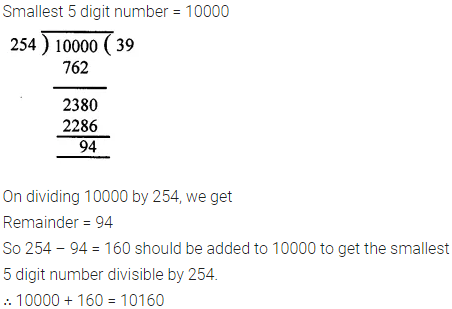Question 14.
A vendor supplies 72 litres of milk to a student’s hostel in the morning and 28 litres of milk in the evening every day. If the milk costs?39 per litre, how much money is due to the vendor per day?
Solution: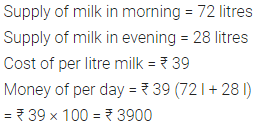Question 15.
State whether the following statements are true (T) or false (F):
(i) If the product of two whole numbers is zero, then atleast
one of them will be zero.
(ii) If the product of two whole numbers is 1, then each of
them must be equal to 1.
(iii) If a and b are whole numbers such that a ≠ 0 and b ≠ 0,
then ab may be zero.
Solution: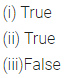Question 16.
Replace each * by the correct digit in each of the following: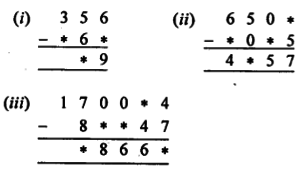Solution: